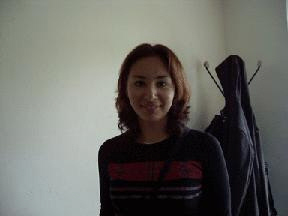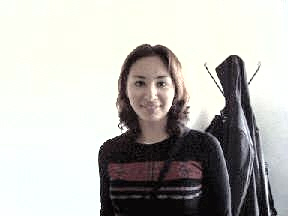﻿ LightControlCommand Class - Namespace: Leadtools.ImageProcessing.Color - Assembly: Leadtools.ImageProcessing.Color - Raster, Medical, Document Help
←Select platform
In This Topic ▼

# LightControlCommand Class

Summary

Lightens or darkens all or part of an image by remapping the pixel values. This function is useful for pre-processing images for the purpose of improving barcode recognition results.

Syntax
C#
VB
Java
Objective-C
C++
``public class LightControlCommand : RasterCommand ``
````Public Class LightControlCommand  `
`   Inherits RasterCommand ````
``@interface LTLightControlCommand : LTRasterCommand ``
``public class LightControlCommand extends RasterCommand ``
``public ref class LightControlCommand : public RasterCommand   ``

Remarks
• This class remaps the pixel values of the image across the full range of available pixel values, in order to achieve the values passed in LowerAverage, Average and UpperAverage. For example, call this method for a grayscale image, with Average containing 150, LowerAverage containing 100 and UpperAverage containing 190. The pixels of the image will be remapped so that the new average pixel value for the entire image will be 150, the new average value for those pixels with a value between 0 and the average value for the entire image will be 100, and the new average value for those pixels with a value between the average value for the entire image and the maximum pixel value in the image will be 190.
• This class supports 12 and 16-bit grayscale and 48 and 64-bit color images. Support for 12 and 16-bit grayscale and 48 and 64-bit color images is available only in the Document/Medical toolkits.
• This class does not support signed data images.
• For an example, see the following figure:• The following figure shows the same image, after the effect has been applied:• To obtain this effect, the following settings were used with the method: LowerAverage = 100 Average = 255 UpperAverage = 255 Type = LightControlCommandType.Yuv

• This command does not support 32-bit grayscale images.

Example

Run the LightControlCommand on an image.

C#
VB
````using Leadtools; `
`using Leadtools.Codecs; `
`using Leadtools.ImageProcessing.Color; `
` `
`public void LightControlCommandExample() `
`{ `
`   // Load an image `
`   RasterCodecs codecs = new RasterCodecs(); `
`   codecs.ThrowExceptionsOnInvalidImages = true; `
` `
`   RasterImage image = codecs.Load(Path.Combine(LEAD_VARS.ImagesDir, "dirty_barcode.jpg")); `
` `
`   // Prepare the command `
`   int[] LowerAverage = new int; `
`   int[] Average = new int; `
`   int[] UpperAverage = new int; `
`   LowerAverage = 100;  //for blue, gray or yuv `
`   LowerAverage = 120;  //for green `
`   LowerAverage = 80;   //for red `
`   Average = 210;       //for blue, gray or yuv `
`   Average = 210;       //for green `
`   Average = 210;       //for red `
`   UpperAverage = 255;  //for blue, gray or yuv `
`   UpperAverage = 255;  //for green `
`   UpperAverage = 255;  //for red  `
`   LightControlCommand command = new LightControlCommand(LowerAverage, Average, UpperAverage, LightControlCommandType.Yuv); `
`   // change the lightness of the image. `
`   command.Run(image); `
`   codecs.Save(image, Path.Combine(LEAD_VARS.ImagesDir, "Result.jpg"), RasterImageFormat.Jpeg, 24); `
` `
`} `
` `
`static class LEAD_VARS `
`{ `
`   public const string ImagesDir = @"C:\Users\Public\Documents\LEADTOOLS Images"; `
`} ````
````Imports Leadtools `
`Imports Leadtools.Codecs `
`Imports Leadtools.ImageProcessing.Color `
` `
`Public Sub LightControlCommandExample() `
`   Dim codecs As New RasterCodecs() `
`   codecs.ThrowExceptionsOnInvalidImages = True `
` `
`   Dim leadImage As RasterImage = codecs.Load(Path.Combine(LEAD_VARS.ImagesDir, "dirty_barcode.jpg")) `
` `
`   ' Prepare the command `
`   Dim LowerAverage() As Integer `
`   ReDim LowerAverage(2) `
`   Dim Average() As Integer `
`   ReDim Average(2) `
`   Dim UpperAverage() As Integer `
`   ReDim UpperAverage(2) `
`   LowerAverage(0) = 100  'for blue, gray or yuv `
`   LowerAverage(1) = 120  'for green `
`   LowerAverage(2) = 80   'for red `
`   Average(0) = 210       'for blue, gray or yuv `
`   Average(1) = 210       'for green `
`   Average(2) = 210       'for red `
`   UpperAverage(0) = 255  'for blue, gray or yuv `
`   UpperAverage(1) = 255  'for green `
`   UpperAverage(2) = 255  'for red  `
`   Dim command As LightControlCommand = New LightControlCommand(LowerAverage, Average, UpperAverage, LightControlCommandType.Yuv) `
`   ' change the lightness of the image. `
`   command.Run(leadImage) `
`   codecs.Save(leadImage, Path.Combine(LEAD_VARS.ImagesDir, "Result.jpg"), RasterImageFormat.Jpeg, 24) `
` `
`End Sub `
` `
`Public NotInheritable Class LEAD_VARS `
`   Public Const ImagesDir As String = "C:\Users\Public\Documents\LEADTOOLS Images" `
`End Class ````
````c#[Silverlight C# Example] `
`using Leadtools; `
`using Leadtools.Codecs; `
`using Leadtools.ImageProcessing.Color; `
`using Leadtools.Examples; `
` `
`public void LightControlCommandExample(RasterImage image, Stream outStream) `
`{ `
`   // Prepare the command `
`   int[] LowerAverage = new int; `
`   int[] Average = new int; `
`   int[] UpperAverage = new int; `
`   LowerAverage = 100;  //for blue, gray or yuv `
`   LowerAverage = 120;  //for green `
`   LowerAverage = 80;   //for red `
`   Average = 150;       //for blue, gray or yuv `
`   Average = 140;       //for green `
`   Average = 128;       //for red `
`   UpperAverage = 190;  //for blue, gray or yuv `
`   UpperAverage = 200;  //for green `
`   UpperAverage = 220;  //for red  `
`   LightControlCommand command = new LightControlCommand(LowerAverage, Average, UpperAverage, LightControlCommandType.Yuv); `
`   // change the lightness of the image. `
`   command.Run(image); `
` `
`   // Save result image `
`   RasterCodecs codecs = new RasterCodecs(); `
`   codecs.Save(image, outStream, RasterImageFormat.Jpeg, 24); `
`   image.Dispose(); `
`} `
`vb[Silverlight VB Example] `
`Imports Leadtools `
`Imports Leadtools.Codecs `
`Imports Leadtools.ImageProcessing.Color `
` `
`Public Sub LightControlCommandExample(ByVal image As RasterImage, ByVal outStream As Stream) `
`   ' Prepare the command `
`   Dim LowerAverage As Integer() = New Integer(2) {} `
`   Dim Average As Integer() = New Integer(2) {} `
`   Dim UpperAverage As Integer() = New Integer(2) {} `
`   LowerAverage(0) = 100 'for blue, gray or yuv `
`   LowerAverage(1) = 120 'for green `
`   LowerAverage(2) = 80 'for red `
`   Average(0) = 150 'for blue, gray or yuv `
`   Average(1) = 140 'for green `
`   Average(2) = 128 'for red `
`   UpperAverage(0) = 190 'for blue, gray or yuv `
`   UpperAverage(1) = 200 'for green `
`   UpperAverage(2) = 220 'for red `
`   Dim command As LightControlCommand = New LightControlCommand(LowerAverage, Average, UpperAverage, LightControlCommandType.Yuv) `
`   ' change the lightness of the image. `
`   command.Run(image) `
` `
`   ' Save result image `
`   Dim codecs As RasterCodecs = New RasterCodecs() `
`   codecs.Save(image, outStream, RasterImageFormat.Jpeg, 24) `
`   image.Dispose() `
`End Sub ````

Requirements

Help Version 20.0.2019.3.11